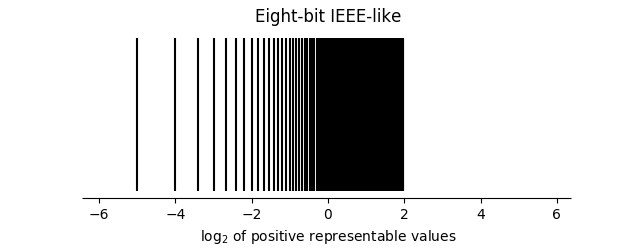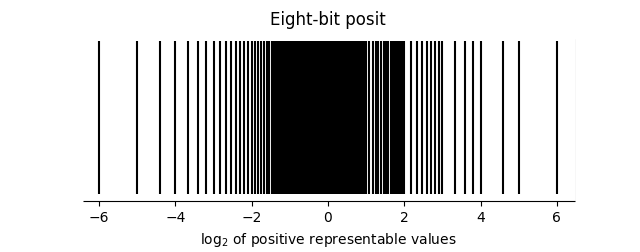# Eight-bit floating point

Researchers have discovered that for some problems, deep neural networks (DNNs) can get by with low precision weights. Using fewer bits to represent weights means that more weights can fit in memory at once. This, as well as embedded systems, has renewed interest in low-precision floating point.

Microsoft mentioned its proprietary floating point formats ms-fp8 and ms-fp9 in connection with its Brainwave Project . I haven’t been able to find any details about these formats, other than that they use two- and three-bit exponents (respectively?).

This post will look at what an 8-bit floating point number would look like if it followed the pattern of IEEE floats or posit numbers. In the notation of the previous post, we’ll look at ieee<8,2> and posit<8,0> numbers. (Update: Added a brief discussion of ieee<8,3>, ieee<8,4>, and posit<8,1> at the end.)

## Eight-bit IEEE-like float

IEEE floating point reserves exponents of all 0’s and all 1’s for special purposes. That’s not as much of a high price with large exponents, but with only four possible exponents, it seems very wasteful to devote half of them for special purposes. Maybe this is where Microsoft does something clever. But for this post, we’ll forge ahead with the analogy to larger IEEE floating point numbers.

There would be 191 representable finite numbers, counting the two representations of 0 as one number. There would be two infinities, positive and negative, and 62 ways to represent NaN.

The smallest non-zero number would be

2-5 = 1/32 = 0.03125.

The largest value would be 01011111 and have value

4(1 – 2-5) = 31/8 = 3.3875.

This makes the dynamic range just over two decades.

## Eight-bit posit

A posit<8, 0> has no significand, just a sign bit, regime, and exponent. But in this case the useed value is 2, and so the range acts like an exponent.

There are 255 representable finite numbers and one value corresponding to ±∞.

The smallest non-zero number would be 1/64 and the largest finite number would be 64. The dynamic range is 3.6 decades.

Update: Here is a list of all possible posit<8,0> numbers.

## Distribution of values

The graphs below give the distribution of 8-bit IEEE-like numbers and 8-bit posits on a log scale.The distribution of IEEE-like numbers is asymmetric because much of the dynamic range comes from denormalized numbers.

The distributions of posits is approximately symmetrical. If a power of 2 is representable as a posit, so is its reciprocal. But you don’t have perfect symmetry because, for example, 3/2 is representable while 2/3 is not.

## Other eight-bit formats

I had originally considered a 2-bit significand because Microsoft’s ms-fp8 format has a two-bit significand. After this post was first published it was suggested in the comments that an ieee<8, 4> float might be better than ieee<8, 2>, so let’s look at that. Let’s look at ieee<8, 3> too while we’re at it. And a posit<8, 1> too.

An ieee<8, 3> floating point number would have a maximum value of 7 and a minimum value of 2-6 = 1/64, a dynamic range of  2.7 decades. It would have 223 finite values, including two zeros, as well as 2 infinities as 30 NaNs.

An ieee<8, 4> floating point number would have a maximum value of 120 and a minimum value of 2-9 = 1/512, a dynamic range of 4.7 decades. It would have 239 finite values, including two zeros, as well as 2 infinities and 14 NaNs.

A posit<8, 1> would have a maximum value of 212 = 4096 and a minimum value of 1/4096, a dynamic range of  7.2 decades. Any 8-bit posit, regardless of the maximum number of exponent bits, will have 255 finite values and one infinity.

Near 1, an ieee<8, 4> has 3 significand bits, an ieee<8, 3> has 4, and a posit<8,1> has 4.

***

 Chung et al. Serving DNNs in Real Time at Datacenter Scale with Project Brainwave. Available here.

## 8 thoughts on “Eight-bit floating point”

1. David W Locke

So the black space is representable and the white space is not?

2. Yes, I plotted the (positive) representable values with a vertical line at each. There are 95 such values for the IEEE-like form and 127 for the posit form.

3. Steve Hollasch

I believe that and IEEE 1:4:3 format (sign:exponent:mantissa) would be a better allocation of bits. This yields signed zero, a range of [1/512, 240], ±∞, six signalling NaNs, and eight quiet NaNs.

4. Steve Hollasch

Oh, the 1:4:3 format would also yield 240 finite numbers, versus 191 with your format.

A four-bit IEEE-like float with two exponent bits would have the following values:

-∞, -3, -2, -3/2, -1, -1/2, -0, +0, 1/2, 1, 3/2, 2, 3, +∞, and two NaNs.

This is pushing IEEE far beyond (below?) what it was designed for.

A posit<4,0> while very limited, makes better use of the 4 bits than the example above. It has values

-4, -2, -3/2, -1, -3/4, -1/2, -1/4, 0, 1/4, 1/2, 3/4, 1, 3/2, 2, 4, and a single value for ±∞.

6. Oren Tirosh

“Those Who Do Not Learn History Are Doomed To Repeat It ”
https://en.wikipedia.org/wiki/G.711

An 8 bit logarithmic encoding with a 14 bit dynamic range. This was standardized in 1972 when every logic gate counted so the hardware implementation must be very efficient.

No infinities, but the extremes might be used for that, if necessary. There are two zeros so one of them could be used as NaN.

Almost every phone call in the world is encoded this way, with the exception of some mobile calls that are compressed end-to-end with some other codec.

7. Steve Hollasch

Re: G.711 — pretty cool. Looks like IEEE FP with 1 sign, 3 exponent , 4 mantissa, 0 exponent bias, no denorm/NaN/inf. Pretty simple. It also has the nice attribute that _x_ + 1 yields the next larger representable number, like IEEE.

8. Jacob Rus

Diagrams like this work a lot better if you use little triangles instead of rectangles.